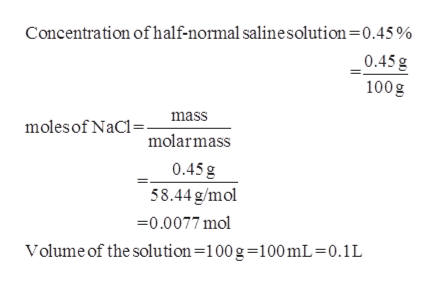Calculate the molarity of sodium chloride in a half‑normal saline solution (0.450.45% NaClNaCl). The molar mass of NaClNaCl is 58.44 g/mol58.44 g/mol.

Question

Calculate the molarity of sodium chloride in a half‑normal saline solution (0.450.45% NaClNaCl). The molar mass of NaClNaCl is 58.44 g/mol58.44 g/mol.

Step 1

The concentration of half normal saline solution is 0.45% and the molar mass of sodium ...help_outlineImage TranscriptioncloseConcentration of half-normal salinesolution=0.45 % 0.45g 100g molesof NaC molarmass mass 0.45 g 58.44g/mol 0.0077 mol Volume of the solution=100g-100 mL = 0.1L fullscreen

Want to see the full answer?

See Solution

Want to see this answer and more?

Our solutions are written by experts, many with advanced degrees, and available 24/7

See Solution
Tagged in

Physical Chemistry